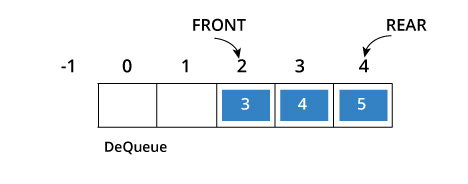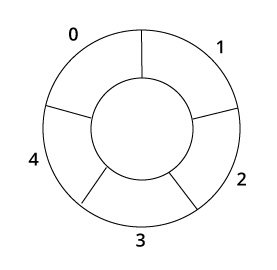# Circular Queue

Circular queue avoids the wastage of space in a regular queue implementation using arrays.As you can see in the above image, after a bit of enqueueing and dequeueing, the size of the queue has been reduced.

The indexes 0 and 1 can only be used after the queue is reset when all the elements have been dequeued.

## How Circular Queue Works

Circular Queue works by the process of circular increment i.e. when we try to increment any variable and we reach the end of queue, we start from the beginning of queue by modulo division with the queue size.

i.e.

`if REAR + 1 == 5 (overflow!), REAR = (REAR + 1)%5 = 0 (start of queue)`Queue operations work as follows:

• Two pointers called FRONT and REAR are used to keep track of the first and last elements in the queue.
• When initializing the queue, we set the value of FRONT and REAR to -1.
• On enqueing an element, we circularly increase the value of REAR index and place the new element in the position pointed to by REAR.
• On dequeueing an element, we return the value pointed to by FRONT and circularly increase the FRONT index.
• Before enqueing, we check if queue is already full.
• Before dequeuing, we check if queue is already empty.
• When enqueing the first element, we set the value of FRONT to 0.
• When dequeing the last element, we reset the values of FRONT and REAR to -1.

However, the check for full queue has a new additional case:

• Case 1: FRONT = 0 && `REAR == SIZE - 1`
• Case 2: `FRONT = REAR + 1`

The second case happens when REAR starts from 0 due to circular increment and when its value is just 1 less than FRONT, the queue is full.## Circular Queue Implementation in programming language

The most common queue implementation is using arrays, but it can also be implemented using lists.

### Implementation using C programming

``````#include <stdio.h>

#define SIZE 5

int items[SIZE];
int front = -1, rear =-1;

int isFull()
{
if( (front == rear + 1) || (front == 0 && rear == SIZE-1)) return 1;
return 0;
}

int isEmpty()
{
if(front == -1) return 1;
return 0;
}

void enQueue(int element)
{
if(isFull()) printf("\n Queue is full!! \n");
else
{
if(front == -1) front = 0;
rear = (rear + 1) % SIZE;
items[rear] = element;
printf("\n Inserted -> %d", element);
}
}

int deQueue()
{
int element;
if(isEmpty()) {
printf("\n Queue is empty !! \n");
return(-1);
} else {
element = items[front];
if (front == rear){
front = -1;
rear = -1;
} /* Q has only one element, so we reset the queue after dequeing it. ? */
else {
front = (front + 1) % SIZE;

}
printf("\n Deleted element -> %d \n", element);
return(element);
}
}

void display()
{
int i;
if(isEmpty()) printf(" \n Empty Queue\n");
else
{
printf("\n Front -> %d ",front);
printf("\n Items -> ");
for( i = front; i!=rear; i=(i+1)%SIZE) {
printf("%d ",items[i]);
}
printf("%d ",items[i]);
printf("\n Rear -> %d \n",rear);
}
}

int main()
{
// Fails because front = -1
deQueue();

enQueue(1);
enQueue(2);
enQueue(3);
enQueue(4);
enQueue(5);

// Fails to enqueue because front == 0 && rear == SIZE - 1
enQueue(6);

display();
deQueue();

display();

enQueue(7);
display();

// Fails to enqueue because front == rear + 1
enQueue(8);

return 0;
}``````

When you run this program, the output will be

```Inserted -> 1
Inserted -> 2
Inserted -> 3
Inserted -> 4
Inserted -> 5
Queue is full!!

Front -> 0
Items -> 1 2 3 4 5
Rear -> 4

Deleted element -> 1

Front -> 1
Items -> 2 3 4 5
Rear -> 4

Inserted -> 7
Front -> 1
Items -> 2 3 4 5 7
Rear -> 0

Queue is full!!```

### Implementation using C++ programming

``````#include <iostream>
#define SIZE 5   /* Size of Circular Queue */

using namespace std;

class Queue {
private:
int items[SIZE], front, rear;

public:
Queue(){
front = -1;
rear = -1;
}

bool isFull(){
if(front == 0 && rear == SIZE - 1){
return true;
}
if(front == rear + 1) {
return true;
}
return false;
}

bool isEmpty(){
if(front == -1) return true;
else return false;
}

void enQueue(int element){
if(isFull()){
cout << "Queue is full";
} else {
if(front == -1) front = 0;
rear = (rear + 1) % SIZE;
items[rear] = element;
cout << endl << "Inserted " << element << endl;
}
}

int deQueue(){
int element;
if(isEmpty()){
cout << "Queue is empty" << endl;
return(-1);
} else {
element = items[front];
if(front == rear){
front = -1;
rear = -1;
} /* Q has only one element, so we reset the queue after deleting it. */
else {
front=(front+1) % SIZE;
}
return(element);
}
}

void display()
{
/* Function to display status of Circular Queue */
int i;
if(isEmpty()) {
cout << endl << "Empty Queue" << endl;
}
else
{
cout << "Front -> " << front;
cout << endl << "Items -> ";
for(i=front; i!=rear;i=(i+1)%SIZE)
cout << items[i];
cout << items[i];
cout << endl << "Rear -> " << rear;
}
}

};

int main()
{
Queue q;

// Fails because front = -1
q.deQueue();

q.enQueue(1);
q.enQueue(2);
q.enQueue(3);
q.enQueue(4);
q.enQueue(5);

// Fails to enqueue because front == 0 && rear == SIZE - 1
q.enQueue(6);

q.display();

int elem = q.deQueue();

if( elem != -1)
cout << endl << "Deleted Element is " << elem;

q.display();

q.enQueue(7);

q.display();

// Fails to enqueue because front == rear + 1
q.enQueue(8);

return 0;
}``````

When you run this program, the output will be

```Queue is empty

Inserted 1

Inserted 2

Inserted 3

Inserted 4

Inserted 5

Queue is full
Front -> 0
Items -> 12345
Rear -> 4
Deleted Element is 1
Front -> 1
Items -> 2345
Rear -> 4
Inserted 7

Front -> 1
Items -> 23457
Rear -> 0
Queue is full```